# How to Find Nth/Second Highest and Lowest Salary in SQLBased on what I have heard from friends and other online users and from my own personal experience, the most common job interview question for database programmers is “How do you find the highest salary in an employee table?”

This question tests a candidate’s knowledge of ranking functions, subqueries, common table expression (CTE) and basic SQL.

We will explore the answer with a series of scenarios and questions asked in relation to finding the highest, lowest, and nth highest salary.

Let’s create an employee table and populate it with some test data.

```IF OBJECT_ID (N'Employee' , N'U' ) IS NOT NULL
DROP TABLE Employee;

CREATE TABLE Employee
(
EmployeeId INT PRIMARY KEY ,
Salary Numeric( 18,2 )
);

Insert into Employee Values ( 101,20000.00 );
Insert into Employee Values ( 102,25000.00 );
Insert into Employee Values ( 103,30000.00 );
Insert into Employee Values ( 104,35000.00 );
Insert into Employee Values ( 105,35000.00 );
Insert into Employee Values ( 106,45000.00 );

SELECT * FROM Employee ;
```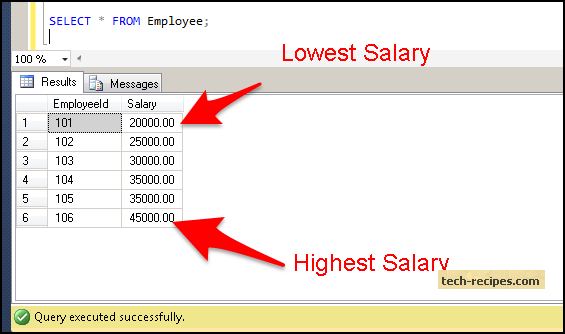Query 1.1 Find the Employee with the Highest Salary

```SELECT EmployeeId, Salary
FROM
(
Select EmployeeId, Salary, ROW_NUMBER() OVER(Order by Salary Desc) as Salary_Order
from   Employee
) DT
WHERE DT. Salary_Order = 1 ;
```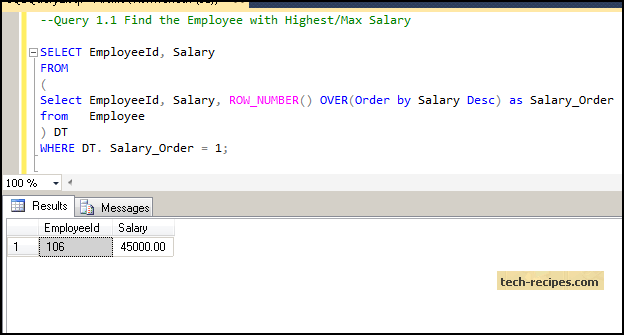The query above uses the derived table concept, where the subquery with row_number ranking function assigns a unique sequential number (1,2,3.. to N) to a salary which is ordered in descending order (highest to lowest). Thus, 1 will be assigned to the highest salary, 2 to the second highest salary, and so on, using the derived table to fetch the row with row_number assigned as 1.

Query 1.2 Find the Employee with the Highest Salary When There Is a Tie (Two employees both have the highest salary and the number is the same)

I am inserting one more employee whose salary matches the highest salary fetched using Query 1.1 to demonstrate this example.

```Insert into Employee Values ( 107,45000.00 );

SELECT EmployeeId, Salary
FROM
(
Select EmployeeId, Salary, DENSE_RANK() OVER(Order by Salary Desc) as Salary_Order
from   Employee
) DT
WHERE DT. Salary_Order = 1 ;
```

Here we are deleting employee id 107 which we had inserted for the Query 1.2 demonstration.

```Delete from employee where EmployeeId = 107;
```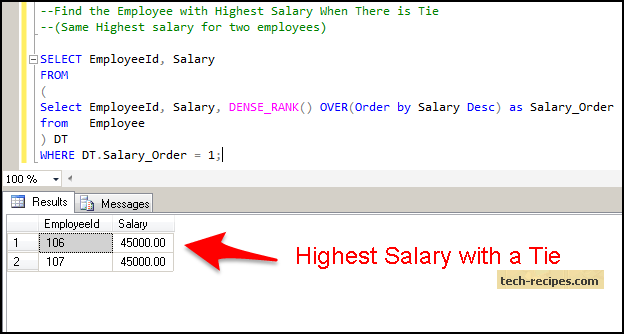In the query above, the Dense_rank function assigns the same consecutive rank number when there is a tie. Therefore, it assigns number 1 to both of the highest salaries (45,000), and both are returned using the derived table query with the salary_order = 1 filter.

Query 1.3 Finding the Employee with the Second Highest Salary

```SELECT EmployeeId, Salary
FROM
(
Select EmployeeId, Salary, ROW_NUMBER() OVER(Order by Salary Desc) as Salary_Order
from   Employee
) DT
WHERE DT. Salary_Order = 2 ;
```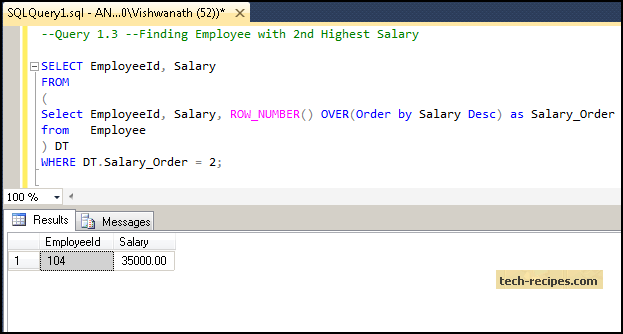Here we are using the same logic used in Query 1.1 with the ROW_NUMBER() function, but we are using Salary_order = 2 to fetch second Highest salary.

Query 1.4 Finding the Employee with the Nth Highest Salary

```SELECT EmployeeId, Salary
FROM
(
Select EmployeeId, Salary, ROW_NUMBER() OVER(Order by Salary Desc) as Salary_Order
from   Employee
) DT
WHERE DT. Salary_Order = 4 ;
```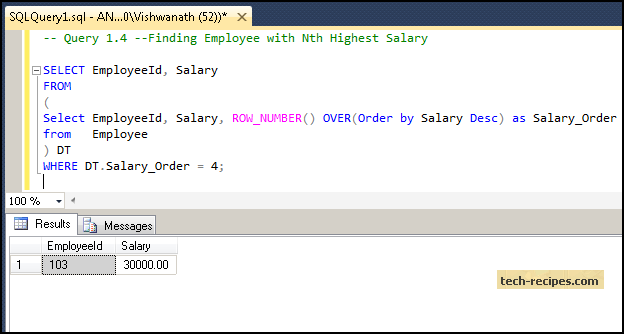Here we are using the same logic used in Query 1.1 and Query 1.3. Nth means you can specify any number, and the query will retrieve the salary at Nth number.

Query 1.5 Finding the Employee with the Lowest Salary

```SELECT EmployeeId, Salary
FROM
(
Select EmployeeId, Salary, ROW_NUMBER() OVER(Order by Salary asc) as Salary_Order
from   Employee
) DT
WHERE DT. Salary_Order = 1 ;
```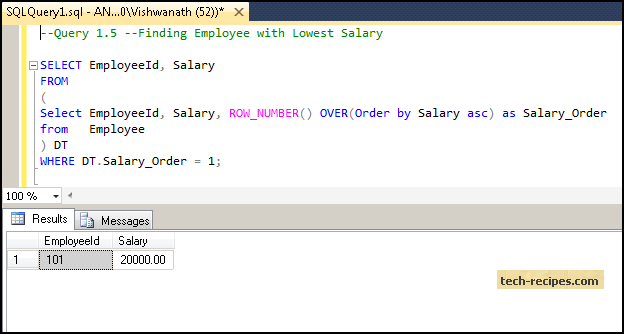To find the lowest salary, we are using Order by salary in ascending order, so the result is sorted in ascending order for salary (lowest to highest). Hence, the lowest salary will get row_number = 1 and so on. We are using the filter Salary_Order = 1 to retrieve the first lowest salary in the employee table.

Query 1.6 Finding the Employee with the Lowest Salary When There Is a Tie (Two employees both have the lowest salary and it is the same)

I am inserting one more employee whose salary matches the lowest salary fetched using the query above to demonstrate this example.

```Insert into Employee Values (109,20000.00);
```

```SELECT EmployeeId, Salary
FROM
(
Select EmployeeId, Salary, DENSE_RANK() OVER(Order by Salary asc) as Salary_Order
from   Employee
) DT
WHERE DT. Salary_Order = 1 ;
```

Here we are deleting the employee with 108 id which was inserted to demonstrate the query above.

```delete from employee where employeeid = 109;
```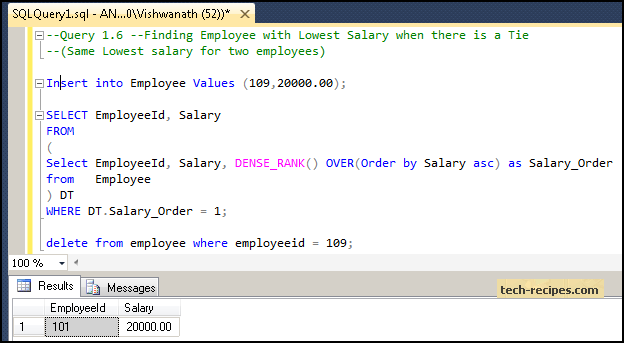To find the lowest salary with ties, we are using the dense_rank function which is the same as Query 1.2. The dense_rank function will assign consecutive numbers where there is a duplicate salary, so for the lowest salary (20000.00), it will assign the number 1 to both the salaries. Using the Salary_Order = 1 filter, we are able to retrieve both the lowest salary when there is tie using the dense_rank function.

Query 1.7 Finding the Employee with the Second Lowest Salary

```SELECT EmployeeId, Salary
FROM
(
Select EmployeeId, Salary, ROW_NUMBER() OVER(Order by Salary asc) as Salary_Order
from  Employee
) DT
WHERE DT. Salary_Order = 2 ;
```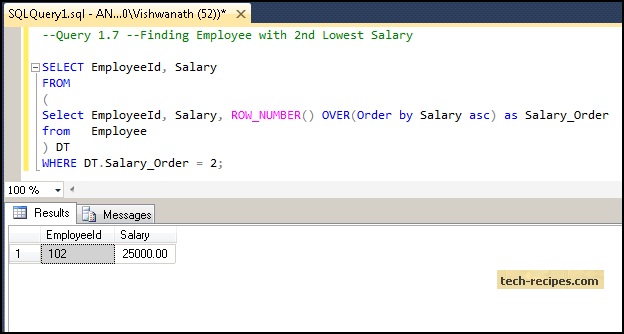Here we are using the same logic used in Query 1.3 with the ROW_NUMBER() function, but we are using Salary_order = 2 to fetch second lowest salary.